# C code to calculate cyclomatic complexity using verteces,edges and connected components

#include<stdio.h>
#include<conio.h>
#include<stdlib.h>
#define MAX 10

void main()
{
FILE *fp;
int row,col,i,j,edges,nodes;
char ch;
int mat[MAX][MAX];

clrscr();

fp=fopen("matrix.txt","r");

row=col=0;

while(fscanf(fp,"%c",&ch)!=EOF)
{
if(ch==' ')
col++;
else if(ch=='\n')
{
row++;
col=0;
}
else
mat[row][col]=ch-48;
}

fclose(fp);

printf("\nThe given adjacency matrix is : \n");

for(i=0;i<=row;i++)
{
for(j=0;j<=col;j++)
printf("%d\t",mat[i][j]);
printf("\n");
}

nodes=row+1;
printf("\nNumber of nodes = %d",nodes);

edges=0;

for(i=0;i<=row;i++)
{
for(j=0;j<=col;j++)
{
if(mat[i][j]==1)
edges++;
}
}
printf("\nNumber of edges = %d",edges);
printf("\n\nCyclometic Complexity of graph  = edges-nodes+2p\n");
printf("\t\t\t\t= %d - %d + 2",edges,nodes);
printf("\n\t\t\t\t= %d",edges-nodes+2);

getch();
}

1.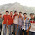useful code....!!what does the matrix.txt file contains???

1.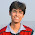Contains the adjacency matrix of the Control Flow Graph of a program.

2.we know that it contains adjacency matrix but we want code.

2.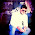the program is not workin

1.Remove clearscreen and instead of void main ...just give int main and run it

2.broo do u have matrix.txt file??

3.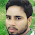This comment has been removed by a blog administrator.

4.where is the adjacent matrix file ???, can anyone share the file

1.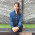yes please where is the file